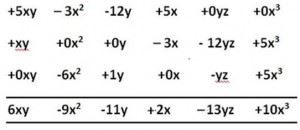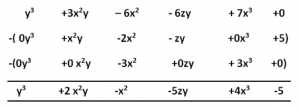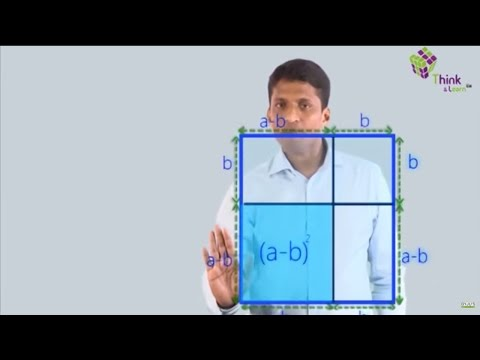# Addition And Subtraction Of Algebraic Expressions

The four basic mathematics operations viz. addition, subtraction, multiplication, and division can also be performed on algebraic equations or expressions. The addition and subtraction of algebraic expressions are almost similar to the addition and subtraction of numbers. However, a combination of variables, constants, and operators constitute an algebraic expression. But in the case of algebraic expressions, like terms and the unlike terms must be sorted together.

## How to Add and Subtract Algebraic Expressions?

The knowledge of like and unlike terms is crucial while studying addition and subtraction of algebraic expressions because the operation of addition and subtraction can only be performed on like terms. The terms whose variables and their exponents are the same are known as like terms and the terms having different variables are unlike terms.

Example: -5x2 + 12 xy – 3y + 7x2 + xy

In the given algebraic expression, -5x2 and 7x2 are like since both the terms have x2 as the common variable. Similarly, 12xy and xy are like terms.

There are two methods to add and subtract algebraic expressions, namely horizontal method and vertical method. Let us understand these two methods along with the examples in detailed steps.

Horizontal Method

Consider three algebraic expressions 5xy – 3x2 – 12y +5x, xy – 3x – 12yz + 5x3 and y – 6x2 – zy + 5x3.

Step 1: Write the given algebraic expressions using an additional symbol.

(5xy – 3x2 – 12y +5x) + (xy – 3x – 12yz + 5x3) + (y – 6x2 – zy + 5x3)

Step 2: Open the brackets and multiply the signs.

5xy – 3x2 – 12y + 5x + xy – 3x – 12yz + 5x3 + y – 6x2 – zy + 5x3

Step 3: Now, combine the like terms.

(5xy + xy) + (-3x2 – 6x2) + (-12y + y) + (5x – 3x) + (-12yz – yz) + (5x3 + 5x3)

Step 4: Add the coefficients. Keep the variables and exponents on the variables the same.

6xy – 9x2 – 11y + 2x – 13yz + 10x3

Column Method

In this method, the given expression must be written column wise one below the other. For adding two or more algebraic expressions the like terms of both the expressions are grouped together. The coefficients of like terms are added together using simple addition techniques and the variable which is common is retained as it is. The, unlike terms, are retained as it is and the result obtained is the addition of two or more algebraic expressions.We can arrange the terms of the given expressions in the same order to represent the result.

## Subtraction of Algebraic Expressions

Similar to the addition of algebraic expressions, we can subtract algebraic expressions in two methods. Let’s understand these two methods along with the example.

Example:

Subtract x2y – 2x2 – zy + 5 and –3x2 + 3x3 from y3 + 3x2y – 6x2 – 6zy + 7x3

Solution:

Let’s solve this problem in two ways.

Horizontal Method:

Step 1: Write the given algebraic expressions using an additional symbol.

(y3 + 3x2y – 6x2 – 6zy + 7x3) – (x2y – 2x2 – zy + 5) – (–3x2 + 3x3)

Step 2: Open the brackets and multiply the signs.

y3 + 3x2y – 6x2 – 6zy + 7x3 – x2y + 2x2 + zy – 5 + 3x2 – 3x3

Step 3: Now, combine the like terms.

y3 + (3x2y – x2y) + (-6x2 + 2x2 + 3x2) + (-6zy + zy) + (7x3 – 3x3) + (-5)

Step 4: Add the coefficients. Keep the variables and exponents on the variables the same.

y3 + 2x2y – x2 – 5zy + 4x3 – 5

Column Method:

For subtracting two or more algebraic expressions, it’s a better practice to write the expressions to be subtracted below the expression from which it is to be subtracted from. Like terms are placed below each other. The sign of each term which is to be subtracted is reversed and then the resulting expression is added normally.In this way, we can add and subtract two or more expressions in either of the methods.

### Practice Problems

1. Add 8a3b3 + 4ab3 – 2a2b3 and 8a2 – a2b3 + 4ab3
2. Subtract 44x4 + 17x3 + x – 18 from 54x4 – 33x3 – 28x2 + 7x – 6.
3. Simplify: (10x2 + 14) + (9x2 + 3) – (8x2 – 6)
4.  Simplify: (29x3 – 11x + 30) – (17x3 – 8x – 22) + (37x2 + 4)